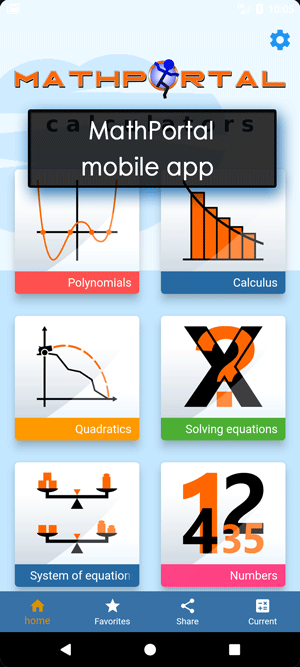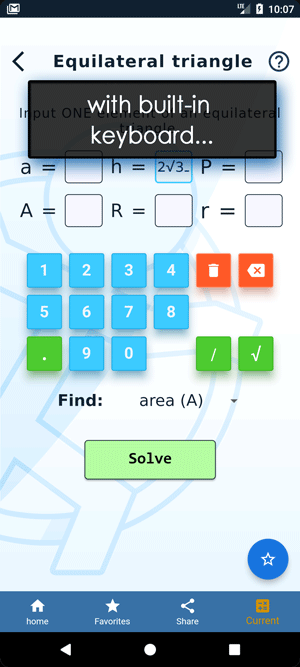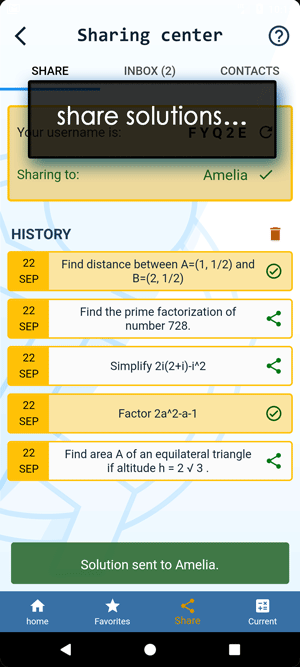Math Calculators, Lessons and Formulas

It is time to solve your math problem

mathportal.org

# Distance between a line and a point calculator

This online calculator can find the distance between a given line and a given point.

Distance between a line and a point
show help ↓↓ examples ↓↓
Hide graph
Hide steps
Find approximate solutionworking...
examples
example 1:ex 1:
Find the distance from the line $3x + 4y - 5 = 0$ to the point point $( -2, 5)$.
example 2:ex 2:
Find the perpendicular distance from the point $(5, -1)$ to the line $y = \frac{1}{2}x + 2$
example 3:ex 3:
Find the perpendicular distance from the point $(-3, 1)$ to the line $y = 2x + 4$.
Search our database of more than 200 calculators